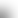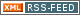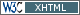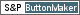www.LinuxHowtos.org howtos, tips&tricks and tutorials for linuxfrom small one page howto to huge articles all in one place

search text in:

Poll
Which screen resolution do you use?

poll results

using iotop to find disk usage hogs

using iotop to find disk usage hogs

words:

887

views:

169775

userrating:

average rating: 1.7 (102 votes) (1=very good 6=terrible)

May 25th. 2007:
Words

486

Views

249260

Workaround and fixes for the current Core Dump Handling vulnerability affected kernels

Workaround and fixes for the current Core Dump Handling vulnerability affected kernels

words:

161

views:

135580

userrating:

average rating: 1.4 (41 votes) (1=very good 6=terrible)

April, 26th. 2006:

You are here: manpages

# EC_GROUP_new

Section: OpenSSL (3)
Updated: 2017-05-25

## NAME

EC_GROUP_new, EC_GROUP_free, EC_GROUP_clear_free, EC_GROUP_new_curve_GFp, EC_GROUP_new_curve_GF2m, EC_GROUP_new_by_curve_name, EC_GROUP_set_curve_GFp, EC_GROUP_get_curve_GFp, EC_GROUP_set_curve_GF2m, EC_GROUP_get_curve_GF2m, EC_get_builtin_curves - Functions for creating and destroying EC_GROUP objects.

## SYNOPSIS

``` #include <openssl/ec.h>
#include <openssl/bn.h>

EC_GROUP *EC_GROUP_new(const EC_METHOD *meth);
void EC_GROUP_free(EC_GROUP *group);
void EC_GROUP_clear_free(EC_GROUP *group);

EC_GROUP *EC_GROUP_new_curve_GFp(const BIGNUM *p, const BIGNUM *a, const BIGNUM *b, BN_CTX *ctx);
EC_GROUP *EC_GROUP_new_curve_GF2m(const BIGNUM *p, const BIGNUM *a, const BIGNUM *b, BN_CTX *ctx);
EC_GROUP *EC_GROUP_new_by_curve_name(int nid);

int EC_GROUP_set_curve_GFp(EC_GROUP *group, const BIGNUM *p, const BIGNUM *a, const BIGNUM *b, BN_CTX *ctx);
int EC_GROUP_get_curve_GFp(const EC_GROUP *group, BIGNUM *p, BIGNUM *a, BIGNUM *b, BN_CTX *ctx);
int EC_GROUP_set_curve_GF2m(EC_GROUP *group, const BIGNUM *p, const BIGNUM *a, const BIGNUM *b, BN_CTX *ctx);
int EC_GROUP_get_curve_GF2m(const EC_GROUP *group, BIGNUM *p, BIGNUM *a, BIGNUM *b, BN_CTX *ctx);

size_t EC_get_builtin_curves(EC_builtin_curve *r, size_t nitems);

```

## DESCRIPTION

Within the library there are two forms of elliptic curve that are of interest. The first form is those defined over the prime field Fp. The elements of Fp are the integers 0 to p-1, where p is a prime number. This gives us a revised elliptic curve equation as follows:

y^2 mod p = x^3 +ax + b mod p

The second form is those defined over a binary field F2^m where the elements of the field are integers of length at most m bits. For this form the elliptic curve equation is modified to:

y^2 + xy = x^3 + ax^2 + b (where b != 0)

Operations in a binary field are performed relative to an irreducible polynomial. All such curves with OpenSSL use a trinomial or a pentanomial for this parameter.

A new curve can be constructed by calling EC_GROUP_new, using the implementation provided by meth (see EC_GFp_simple_method(3)). It is then necessary to call either EC_GROUP_set_curve_GFp or EC_GROUP_set_curve_GF2m as appropriate to create a curve defined over Fp or over F2^m respectively.

EC_GROUP_set_curve_GFp sets the curve parameters p, a and b for a curve over Fp stored in group. EC_group_get_curve_GFp obtains the previously set curve parameters.

EC_GROUP_set_curve_GF2m sets the equivalent curve parameters for a curve over F2^m. In this case p represents the irreducible polybnomial - each bit represents a term in the polynomial. Therefore there will either be three or five bits set dependant on whether the polynomial is a trinomial or a pentanomial. EC_group_get_curve_GF2m obtains the previously set curve parameters.

The functions EC_GROUP_new_curve_GFp and EC_GROUP_new_curve_GF2m are shortcuts for calling EC_GROUP_new and the appropriate EC_group_set_curve function. An appropriate default implementation method will be used.

Whilst the library can be used to create any curve using the functions described above, there are also a number of predefined curves that are available. In order to obtain a list of all of the predefined curves, call the function EC_get_builtin_curves. The parameter r should be an array of EC_builtin_curve structures of size nitems. The function will populate the r array with information about the builtin curves. If nitems is less than the total number of curves available, then the first nitems curves will be returned. Otherwise the total number of curves will be provided. The return value is the total number of curves available (whether that number has been populated in r or not). Passing a NULL r, or setting nitems to 0 will do nothing other than return the total number of curves available. The EC_builtin_curve structure is defined as follows:

``` typedef struct {
int nid;
const char *comment;
} EC_builtin_curve;

```

Each EC_builtin_curve item has a unique integer id (nid), and a human readable comment string describing the curve.

In order to construct a builtin curve use the function EC_GROUP_new_by_curve_name and provide the nid of the curve to be constructed.

EC_GROUP_free frees the memory associated with the EC_GROUP.

EC_GROUP_clear_free destroys any sensitive data held within the EC_GROUP and then frees its memory.

## RETURN VALUES

All EC_GROUP_new* functions return a pointer to the newly constructed group, or NULL on error.

EC_get_builtin_curves returns the number of builtin curves that are available.

EC_GROUP_set_curve_GFp, EC_GROUP_get_curve_GFp, EC_GROUP_set_curve_GF2m, EC_GROUP_get_curve_GF2m return 1 on success or 0 on error.

crypto(3), ec(3), EC_GROUP_copy(3), EC_POINT_new(3), EC_POINT_add(3), EC_KEY_new(3), EC_GFp_simple_method(3), d2i_ECPKParameters(3)

## Index

NAME
SYNOPSIS
DESCRIPTION
RETURN VALUES||- Powered by- Running on-
Copyright 2004-2020 Sascha Nitsch Unternehmensberatung GmbH::- Level Triple-A Conformance to Web Content Accessibility Guidelines 1.0 -
- Copyright and legal notices -